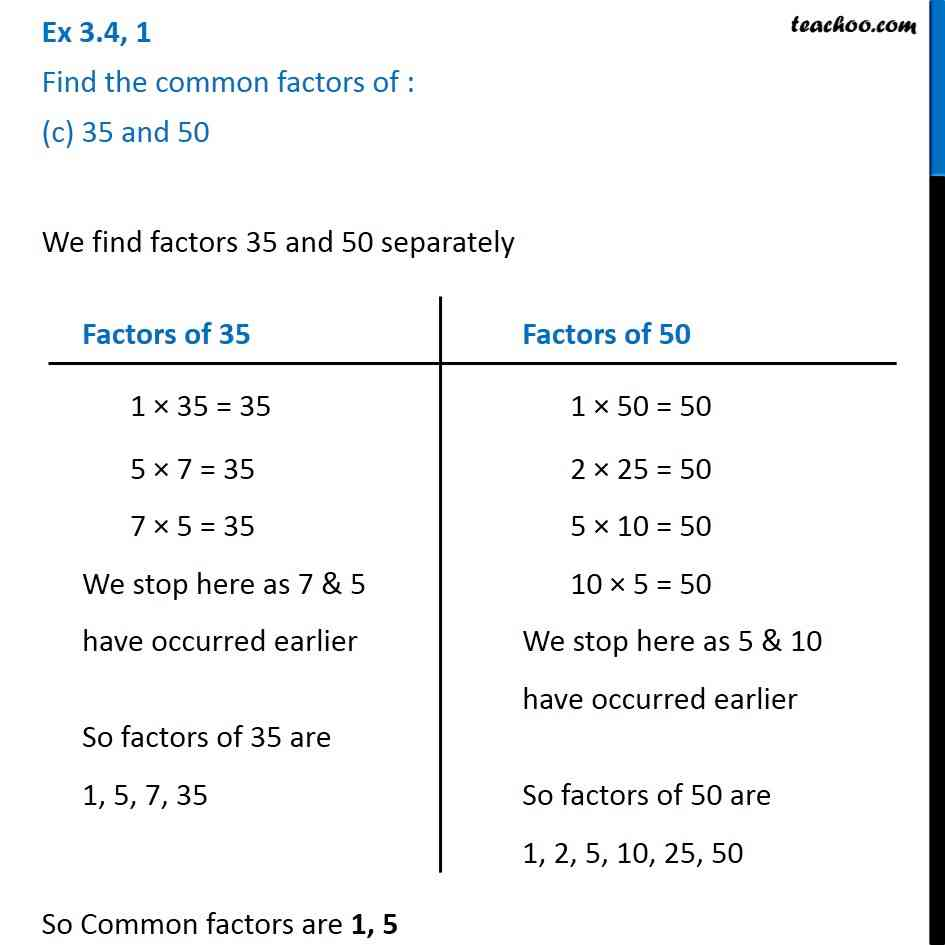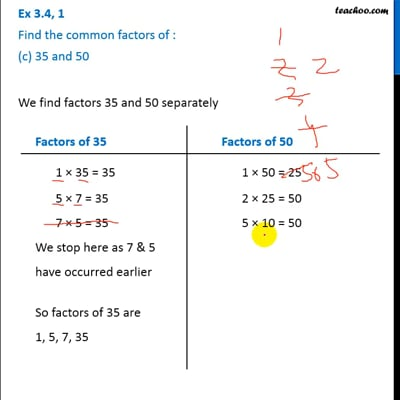Ex 3.4

Chapter 3 Class 6 Playing with Numbers
Serial order wiseThis video is only available for Teachoo black users

### Transcript

Ex 3.4, 1 Find the common factors of : (c) 35 and 50 We find factors 35 and 50 separately So Common factors are 1, 5 Factors of 35 1 × 35 = 35 5 × 7 = 35 7 × 5 = 35 We stop here as 7 & 5 have occurred earlier So factors of 35 are 1, 5, 7, 35 Factors of 50 1 × 50 = 50 2 × 25 = 50 5 × 10 = 50 10 × 5 = 50 We stop here as 5 & 10 have occurred earlier So factors of 50 are 1, 2, 5, 10, 25, 50